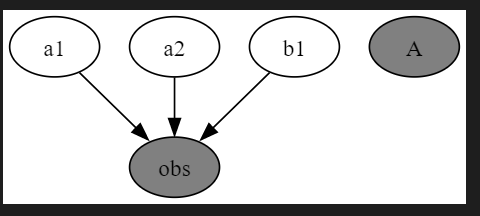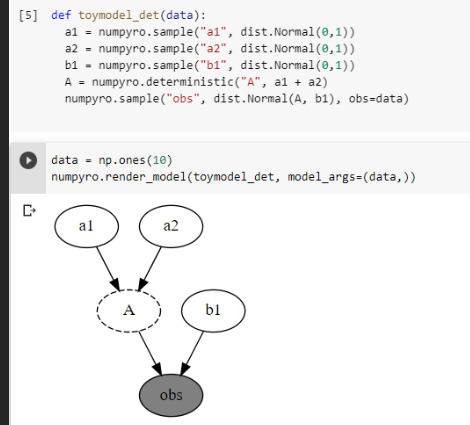# Deterministic not rendered as nodes with Pyro

I’m using pyro to build and render a model, but I think that deterministic relationships are not rendered correctly.
Using pyro, the deterministic object is visualized as an independent observed object, whereas I would expect to see it as an internal node.
This is a simple model to show what I mean

``````def toymodel_det(data):
a1 = pyro.sample("a1", dist.Normal(0, 1))
a2 = pyro.sample('a2',dist.Beta(0,5))
b1 = pyro.sample('b1',dist.Beta(0,5))
A = pyro.deterministic('A', a1+a2 )
pyro.sample("obs", dist.Normal(A, b1), obs=data)

data = torch.ones(10)
pyro.render_model(toymodel_det, model_args=(data,))
``````

and this plotsUsing numpyro, instead, and using the same model, it yields (screen of the code as well)which is what I would expect.
Is there a way to get deterministic relationships shown also with pyro?

Hi @NM_user, we haven’t implemented rendering deterministic sites yet in Pyro. Here’s the issue where we’re tracking work: